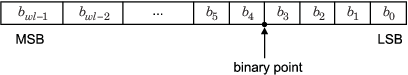Documentation

## Fixed-Point Data Types

In digital hardware, numbers are stored in binary words. A binary word is a fixed-length sequence of bits (1's and 0's). How hardware components or software functions interpret this sequence of 1's and 0's is defined by the data type.

Binary numbers are represented as either fixed-point or floating-point data types. This chapter discusses many terms and concepts relating to fixed-point numbers, data types, and mathematics.

A fixed-point data type is characterized by the word length in bits, the position of the binary point, and whether it is signed or unsigned. The position of the binary point is the means by which fixed-point values are scaled and interpreted.

For example, a binary representation of a generalized fixed-point number (either signed or unsigned) is shown below:where

• bi is the ith binary digit.

• wl is the word length in bits.

• bwl-1 is the location of the most significant, or highest, bit (MSB).

• b0 is the location of the least significant, or lowest, bit (LSB).

• The binary point is shown four places to the left of the LSB. In this example, therefore, the number is said to have four fractional bits, or a fraction length of four.

Fixed-point data types can be either signed or unsigned. Signed binary fixed-point numbers are typically represented in one of three ways:

Two's complement is the most common representation of signed fixed-point numbers and is the only representation used by Fixed-Point Designer™ documentation.

##### Support평가판 신청